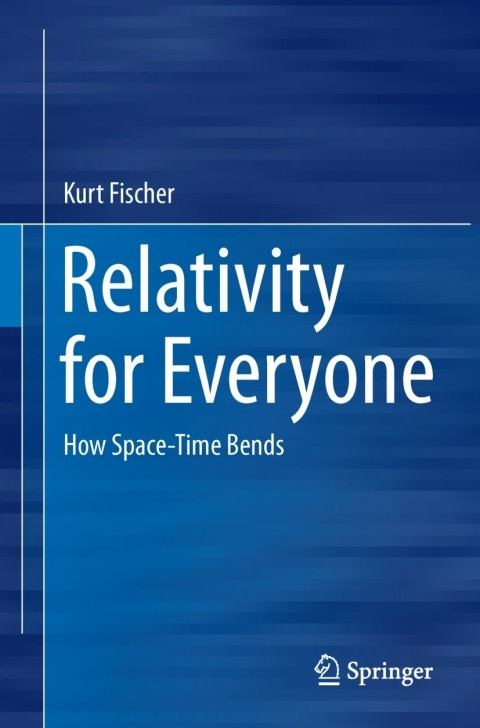• Author(s) Kurt Fischer
• Edition
• Published07052013
• PublisherSpringer Nature
• ISBN9783319005874
This book explains the theory of special and general relativity in detail, without digressions such as information on Einstein's life or the historical background. However, complicated calculations are replaced with figures and thought experiments, the text being formulated in such a way that the reader will be able to understand the gist intuitively. The first part of the book focuses on the essentials of special relativity. Explanations are provided of the famous equivalence between mass and energy and of why Einstein was able to use the theory of electrodynamics as a template for his "electrodynamics of moving bodies", simply because besides the speed of light, the electric charge itself is also absolute, leading to the relativity of other physical quantities. General relativity is then introduced, mainly with the help of thought experiments. Reference is made to the previously introduced special relativity and the equivalence principle and, using many figures, it is explained how space-time is bending under gravity. The climax of the book comes with the Einstein equations of gravity that describe the way in which matter bends space-time. The reader is shown how to obtain the famous Schwarzschild solution. There follows a numerically correct and yet intuitive explanation of the classic effects such as light bending or the movement of the perihelion. The book concludes by explaining the Friedmann model of the big bang and why the theory of gravity does not fit with quantum theory.

# Relativity for Everyone

##### Get it instantly

Zookal account needed

\$47.52 \$50.00 Save \$2.48
• Author(s) Kurt Fischer
• Edition
• Published07052013
• PublisherSpringer Nature
• ISBN9783319005874
This book explains the theory of special and general relativity in detail, without digressions such as information on Einstein's life or the historical background. However, complicated calculations are replaced with figures and thought experiments, the text being formulated in such a way that the reader will be able to understand the gist intuitively. The first part of the book focuses on the essentials of special relativity. Explanations are provided of the famous equivalence between mass and energy and of why Einstein was able to use the theory of electrodynamics as a template for his "electrodynamics of moving bodies", simply because besides the speed of light, the electric charge itself is also absolute, leading to the relativity of other physical quantities. General relativity is then introduced, mainly with the help of thought experiments. Reference is made to the previously introduced special relativity and the equivalence principle and, using many figures, it is explained how space-time is bending under gravity. The climax of the book comes with the Einstein equations of gravity that describe the way in which matter bends space-time. The reader is shown how to obtain the famous Schwarzschild solution. There follows a numerically correct and yet intuitive explanation of the classic effects such as light bending or the movement of the perihelion. The book concludes by explaining the Friedmann model of the big bang and why the theory of gravity does not fit with quantum theory.